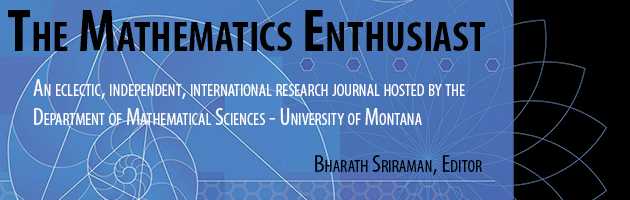•
•21

1-2

#### Abstract

Separation of variables is a common method for producing an analytical based solution to partial differential equations. Despite the wide application of this method, often the physical phenomena described by the differential equations are not adequately involved in the discourse over the appropriate methods to solve a given problem, particularly in mathematics curricula. However, as mathematics is the tool to better understanding of the physical world, the meaning of the differential equation, boundary conditions, and initial conditions cannot be detached from the methods used to solve the differential equations. Failure to recognize the physical conditions being studied can lead to solution methods that are invalid or unphysical. This paper demonstrates how awareness of the physical nature of the system being investigated and its relationship to the mathematics can guide the selection of the relevant solution methods. To illustrate the importance of the comprehension of the physical meaning behind the mathematical equations and representations and the need to avoid rote application a solution technique, the logic behind the selection of the appropriate solution techniques for the one-dimensional transient heat conduction equation is considered under different imposed conditions which lead to different trends in system operation.

301

314

#### Digital Object Identifier (DOI)

10.54870/1551-3440.1627

#### Publisher

University of Montana, Maureen and Mike Mansfield Library

COinS